# 1.4 Inverse functions  (Page 2/11)

 Page 2 / 11

## Rule: horizontal line test

A function $f$ is one-to-one if and only if every horizontal line intersects the graph of $f$ no more than once.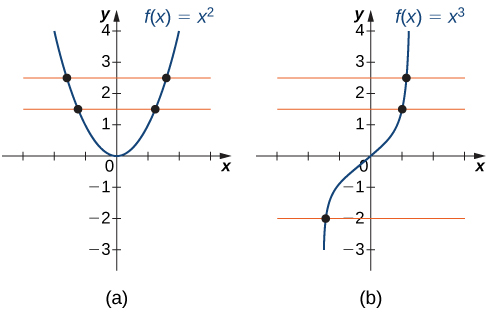(a) The function f ( x ) = x 2 is not one-to-one because it fails the horizontal line test. (b) The function f ( x ) = x 3 is one-to-one because it passes the horizontal line test.

## Determining whether a function is one-to-one

For each of the following functions, use the horizontal line test to determine whether it is one-to-one.

1.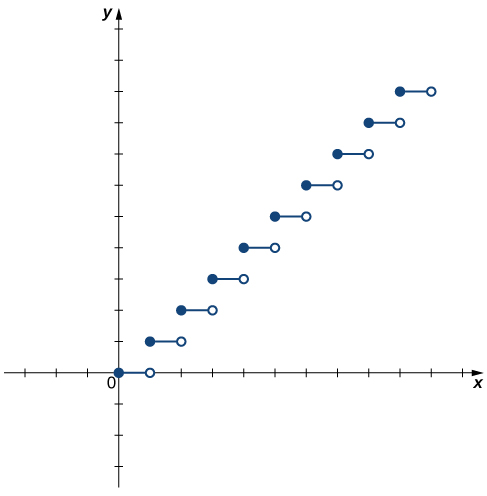2.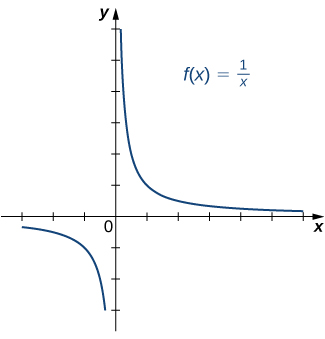1. Since the horizontal line $y=n$ for any integer $n\ge 0$ intersects the graph more than once, this function is not one-to-one.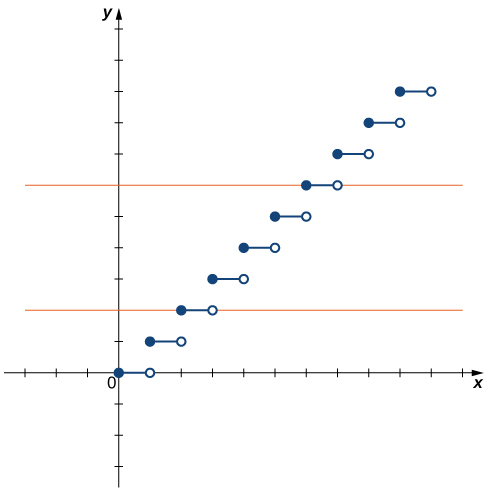2. Since every horizontal line intersects the graph once (at most), this function is one-to-one.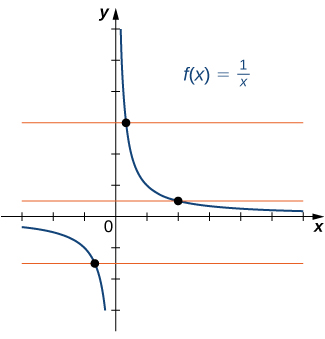Is the function $f$ graphed in the following image one-to-one?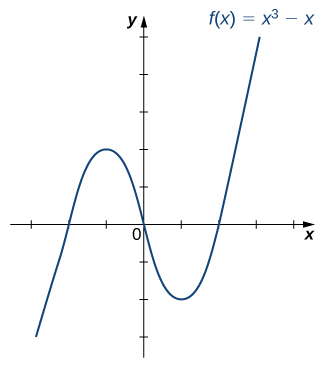No.

## Finding a function’s inverse

We can now consider one-to-one functions and show how to find their inverses. Recall that a function maps elements in the domain of $f$ to elements in the range of $f.$ The inverse function maps each element from the range of $f$ back to its corresponding element from the domain of $f.$ Therefore, to find the inverse function of a one-to-one function $f,$ given any $y$ in the range of $f,$ we need to determine which $x$ in the domain of $f$ satisfies $f\left(x\right)=y.$ Since $f$ is one-to-one, there is exactly one such value $x.$ We can find that value $x$ by solving the equation $f\left(x\right)=y$ for $x.$ Doing so, we are able to write $x$ as a function of $y$ where the domain of this function is the range of $f$ and the range of this new function is the domain of $f.$ Consequently, this function is the inverse of $f,$ and we write $x={f}^{-1}\left(y\right).$ Since we typically use the variable $x$ to denote the independent variable and $y$ to denote the dependent variable, we often interchange the roles of $x$ and $y,$ and write $y={f}^{-1}\left(x\right).$ Representing the inverse function in this way is also helpful later when we graph a function $f$ and its inverse ${f}^{-1}$ on the same axes.

## Problem-solving strategy: finding an inverse function

1. Solve the equation $y=f\left(x\right)$ for $x.$
2. Interchange the variables $x$ and $y$ and write $y={f}^{-1}\left(x\right).$

## Finding an inverse function

Find the inverse for the function $f\left(x\right)=3x-4.$ State the domain and range of the inverse function. Verify that ${f}^{-1}\left(f\left(x\right)\right)=x.$

Follow the steps outlined in the strategy.

Step 1. If $y=3x-4,$ then $3x=y+4$ and $x=\frac{1}{3}y+\frac{4}{3}.$

Step 2. Rewrite as $y=\frac{1}{3}x+\frac{4}{3}$ and let $y={f}^{-1}\left(x\right).$

Therefore, ${f}^{-1}\left(x\right)=\frac{1}{3}x+\frac{4}{3}.$

Since the domain of $f$ is $\left(\text{−}\infty ,\infty \right),$ the range of ${f}^{-1}$ is $\left(\text{−}\infty ,\infty \right).$ Since the range of $f$ is $\left(\text{−}\infty ,\infty \right),$ the domain of ${f}^{-1}$ is $\left(\text{−}\infty ,\infty \right).$

You can verify that ${f}^{-1}\left(f\left(x\right)\right)=x$ by writing

${f}^{-1}\left(f\left(x\right)\right)={f}^{-1}\left(3x-4\right)=\frac{1}{3}\left(3x-4\right)+\frac{4}{3}=x-\frac{4}{3}+\frac{4}{3}=x.$

Note that for ${f}^{-1}\left(x\right)$ to be the inverse of $f\left(x\right),$ both ${f}^{-1}\left(f\left(x\right)\right)=x$ and $f\left({f}^{-1}\left(x\right)\right)=x$ for all x in the domain of the inside function.

Find the inverse of the function $f\left(x\right)=3x\text{/}\left(x-2\right).$ State the domain and range of the inverse function.

${f}^{-1}\left(x\right)=\frac{2x}{x-3}.$ The domain of ${f}^{-1}$ is $\left\{x|x\ne 3\right\}.$ The range of ${f}^{-1}$ is $\left\{y|y\ne 2\right\}.$

## Graphing inverse functions

Let’s consider the relationship between the graph of a function $f$ and the graph of its inverse. Consider the graph of $f$ shown in [link] and a point $\left(a,b\right)$ on the graph. Since $b=f\left(a\right),$ then ${f}^{-1}\left(b\right)=a.$ Therefore, when we graph ${f}^{-1},$ the point $\left(b,a\right)$ is on the graph. As a result, the graph of ${f}^{-1}$ is a reflection of the graph of $f$ about the line $y=x.$

f(x) = x-2 g(x) = 3x + 5 fog(x)? f(x)/g(x)
fog(x)= f(g(x)) = x-2 = 3x+5-2 = 3x+3 f(x)/g(x)= x-2/3x+5
diron
pweding paturo nsa calculus?
jimmy
how to use fundamental theorem to solve exponential
find the bounded area of the parabola y^2=4x and y=16x
what is absolute value means?
Chicken nuggets
Hugh
🐔
MM
🐔🦃 nuggets
MM
(mathematics) For a complex number a+bi, the principal square root of the sum of the squares of its real and imaginary parts, √a2+b2 . Denoted by | |. The absolute value |x| of a real number x is √x2 , which is equal to x if x is non-negative, and −x if x is negative.
Ismael
find integration of loge x
find the volume of a solid about the y-axis, x=0, x=1, y=0, y=7+x^3
how does this work
Can calculus give the answers as same as other methods give in basic classes while solving the numericals?
log tan (x/4+x/2)
Rohan
Rohan
y=(x^2 + 3x).(eipix)
Claudia
Ismael
A Function F(X)=Sinx+cosx is odd or even?
neither
David
Neither
Lovuyiso
f(x)=1/1+x^2 |=[-3,1]
apa itu?
fauzi
determine the area of the region enclosed by x²+y=1,2x-y+4=0
Hi
MP
Hi too
Vic
hello please anyone with calculus PDF should share
Which kind of pdf do you want bro?
Aftab
hi
Abdul
can I get calculus in pdf
Abdul
explain for me
Usman
okay I have such documents
Fitzgerald
Hamza
How to use it to slove fraction
Hello please can someone tell me the meaning of this group all about, yes I know is calculus group but yet nothing is showing up
Shodipo
You have downloaded the aplication Calculus Volume 1, tackling about lessons for (mostly) college freshmen, Calculus 1: Differential, and this group I think aims to let concerns and questions from students who want to clarify something about the subject. Well, this is what I guess so.
Jean
Im not in college but this will still help
nothing
how en where can u apply it
Migos
how can we scatch a parabola graph
Ok
Endalkachew
how can I solve differentiation?
with the help of different formulas and Rules. we use formulas according to given condition or according to questions
CALCULUS
For example any questions...
CALCULUS
v=(x,y) وu=(x,y ) ∂u/∂x* ∂x/∂u +∂v/∂x*∂x/∂v=1
log tan (x/4+x/2)
Rohan
what is the procedures in solving number 1?

#### Get Jobilize Job Search Mobile App in your pocket Now!By OpenStaxBy Laurence BailenBy OpenStaxBy Brooke DelaneyBy David MartinBy Marriyam RanaBy Dewey ComptonBy Richley CrapoBy Anindyo MukhopadhyayBy OpenStax